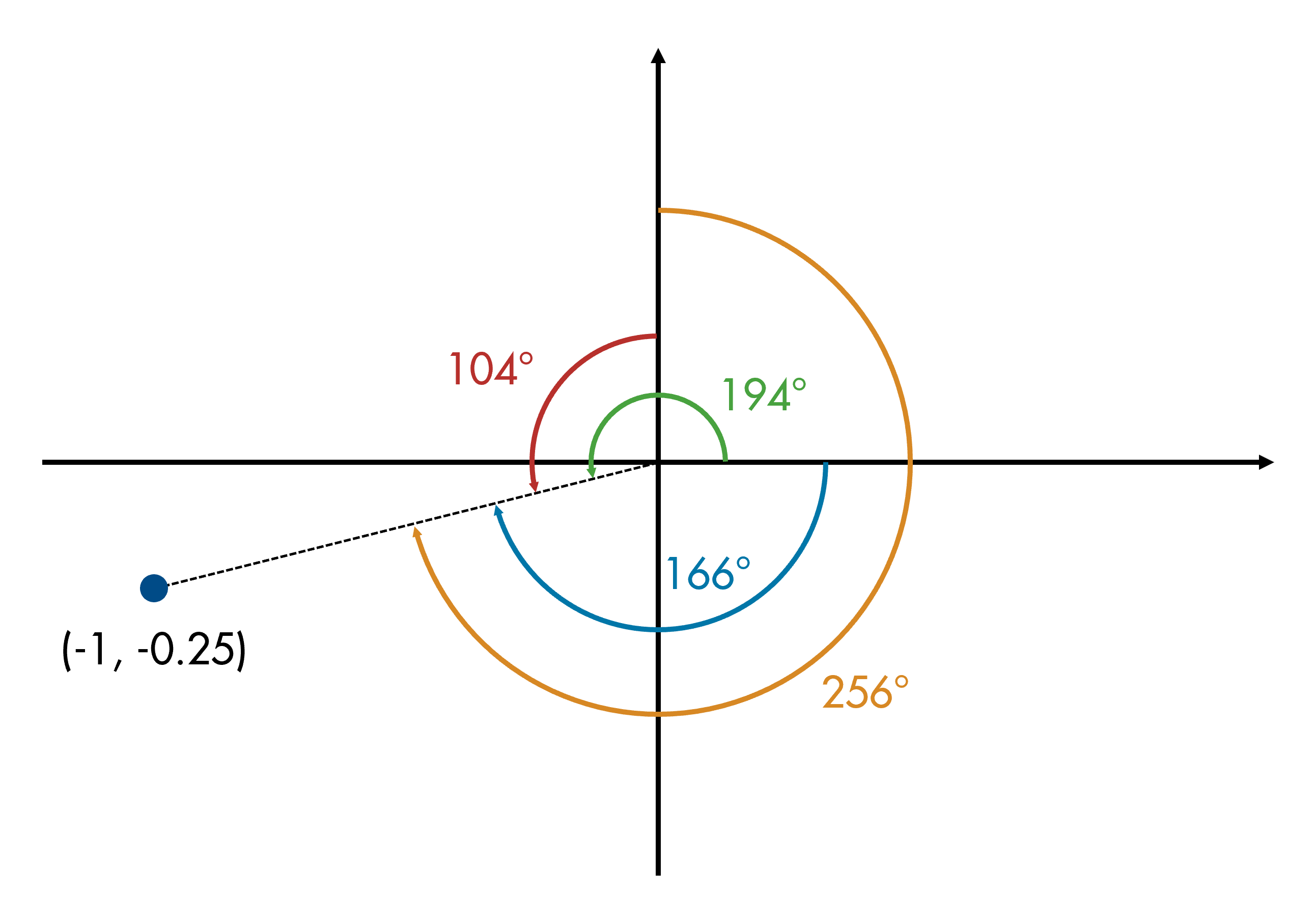# Problem 55780. Calculate Angle From Axis

Given coordinates x and y, an axis ("X" or "Y"), and a direction ("cw" or "ccw", meaning clockwise and counterclockwise, respectively), find the angle, in degrees, from the given axis to the vector from the origin to the point (x,y), in the given direction. The angle must be given in the range [0,360].
You can assume that the axis and direction will always be in the form given above ("X" or "Y" for the axis, "cw" or "ccw" for the direction).
For example:theta = fromaxis(-1,-0.25,"X","cw") % blue angle in diagram
theta =
165.9638
theta = fromaxis(-1,-0.25,"Y","cw") % orange
theta =
255.9638
theta = fromaxis(-1,-0.25,"X","ccw") % green
theta =
194.0362
theta = fromaxis(-1,-0.25,"Y","ccw") % red
theta =
104.0362
You can use the following algorithm:
1. Use theta = atan2d(y,x) to get the angle counterclockwise from the x axis in the range [-180, 180].
2. Use -theta to get the angle clockwise from the x axis (still in the range [-180, 180]).
3. Use theta - 90 to get the angle counterclockwise from the y axis (in the range [-270, 90]).
4. Use 90 - theta to get the angle clockwise from the y axis (in the range [-90, 270]).
5. Put the resulting angle into the range [0 360].
One way to achieve #5 is to add 360 to any result that is negative.

### Solution Stats

30.4% Correct | 69.6% Incorrect
Last Solution submitted on Nov 27, 2023

### Community Treasure Hunt

Find the treasures in MATLAB Central and discover how the community can help you!

Start Hunting!